Note: The other languages of the website are Google-translated. Back to English

## How to move entire row to another sheet based on cell value in Excel?

For moving entire row to another sheet based on cell value, this article will help you.

Move entire row to another sheet based on cell value with VBA code
Move entire row to another sheet based on cell value with Kutools for Excel

#### Move entire row to another sheet based on cell value with VBA code

As below screenshot shown, you need to move the entire row from Sheet1 to Sheet2 if a specific word “Done” exists in column C. You can try the following VBA code.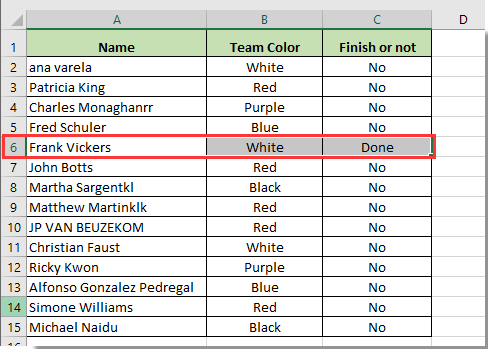1. Press Alt+ F11 keys simultaneously to open the Microsoft Visual Basic for Applications window.

2. In the Microsoft Visual Basic for Applications window, click Insert > Module. Then copy and paste the below VBA code into the window.

VBA code 1: Move entire row to another sheet based on cell value

``````Sub Cheezy()
'Updated by Kutools for Excel 2017/8/28
Dim xRg As Range
Dim xCell As Range
Dim I As Long
Dim J As Long
Dim K As Long
I = Worksheets("Sheet1").UsedRange.Rows.Count
J = Worksheets("Sheet2").UsedRange.Rows.Count
If J = 1 Then
If Application.WorksheetFunction.CountA(Worksheets("Sheet2").UsedRange) = 0 Then J = 0
End If
Set xRg = Worksheets("Sheet1").Range("C1:C" & I)
On Error Resume Next
Application.ScreenUpdating = False
For K = 1 To xRg.Count
If CStr(xRg(K).Value) = "Done" Then
xRg(K).EntireRow.Copy Destination:=Worksheets("Sheet2").Range("A" & J + 1)
xRg(K).EntireRow.Delete
If CStr(xRg(K).Value) = "Done" Then
K = K - 1
End If
J = J + 1
End If
Next
Application.ScreenUpdating = True
End Sub``````

Note: In the code, Sheet1 is the worksheet contains the row you want to move. And Sheet2 is the destination worksheet where you will locate the row to. “C:C” is the column contains the certain value, and the word “Done” is the certain value you will move row based on. Please change them based on your needs.

3. Press the F5 key to run the code, then the row which meet the criteria in Sheet1 will be moved to Sheet2 immediately.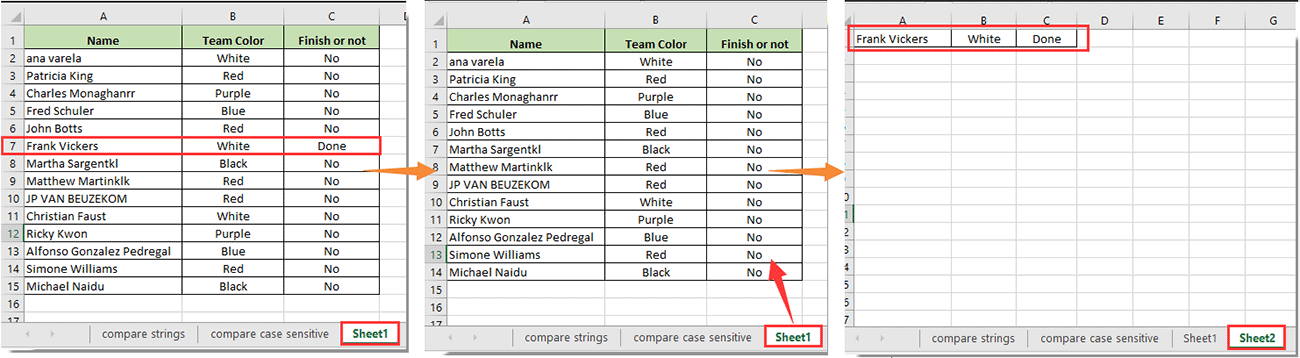Note: The above VBA code will delete rows from the original data after moving to a specified worksheet. If you only want to copy rows based on cell value instead of deleting them. Please apply the below VBA code 2.

VBA code 2: Copy entire row to another sheet based on cell value

``````Sub MoveRowBasedOnCellValue()
'Updated by Extendoffice 2017/11/10
Dim xRg As Range
Dim xCell As Range
Dim I As Long
Dim J As Long
Dim K As Long
I = Worksheets("Sheet1").UsedRange.Rows.Count
J = Worksheets("Sheet2").UsedRange.Rows.Count
If J = 1 Then
If Application.WorksheetFunction.CountA(Worksheets("Sheet2").UsedRange) = 0 Then J = 0
End If
Set xRg = Worksheets("Sheet1").Range("C1:C" & I)
On Error Resume Next
Application.ScreenUpdating = False
For K = 1 To xRg.Count
If CStr(xRg(K).Value) = "Done" Then
xRg(K).EntireRow.Copy Destination:=Worksheets("Sheet2").Range("A" & J + 1)
J = J + 1
End If
Next
Application.ScreenUpdating = True
End Sub``````

#### Move entire row to another sheet based on cell value with Kutools for Excel

If you are newbie in VBA code. Here I introduce the Select Specific Cells utility of Kutools for Excel. With this utility, you can easily select all rows based on a certain cell value or different cell values in a worksheet, and the copy the selected rows to the destination worksheet as you need. Please do as follows.

Before applying Kutools for Excel, please download and install it firstly.

1. Select the column list contains the cell value you will move rows based on, then click Kutools > Select > Select Specific Cells. See screenshot: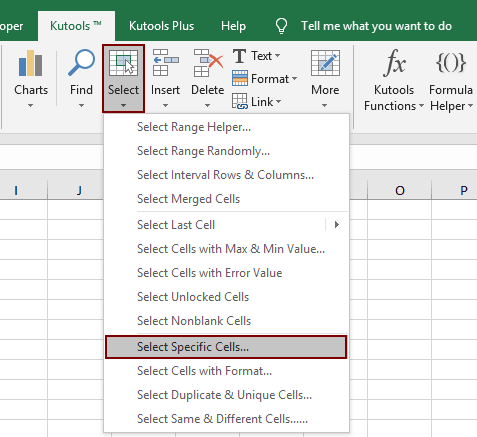2. In the opening Select Specific Cells dialog box, choose Entire row in the Selection type section, select Equals in the Specific type drop-down list, enter the cell value into the text box and then click the OK button.

Another Select Specific Cells dialog box pops up to show you number of selected rows, and meanwhile, all rows contain the specified value in selected column have been selected. See screenshot: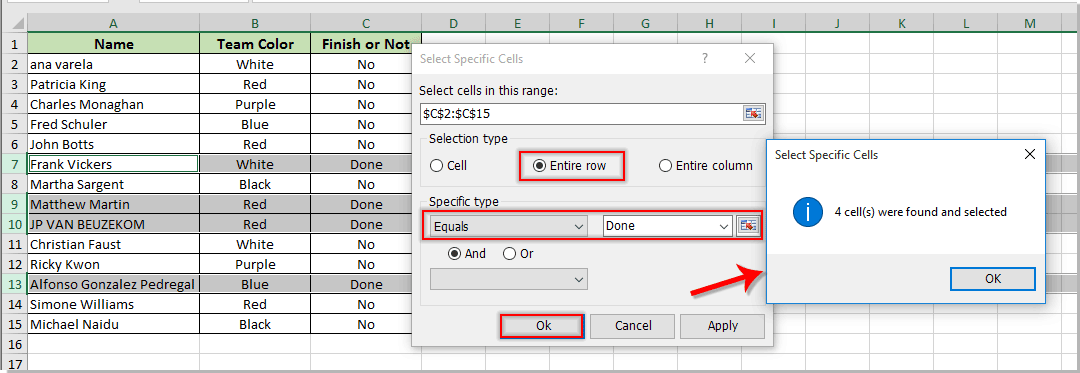3. Press the Ctrl + C keys to copy the selected rows, and then paste them into the destination worksheet you need.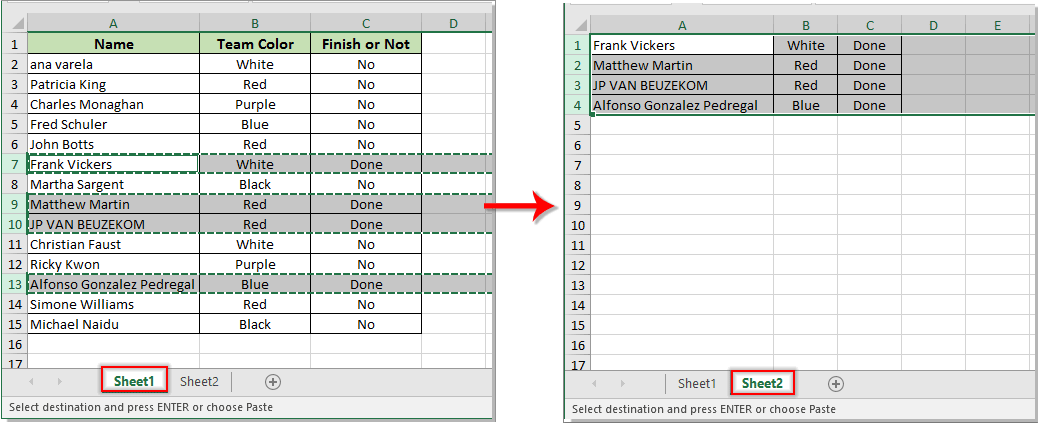Note: If you want to move rows to another worksheet based on two different cell values. For example, move rows based on cell values either "Done" or "Processing", you can enable the Or condition in the Select Specific Cells dialog box as below screenshot shown: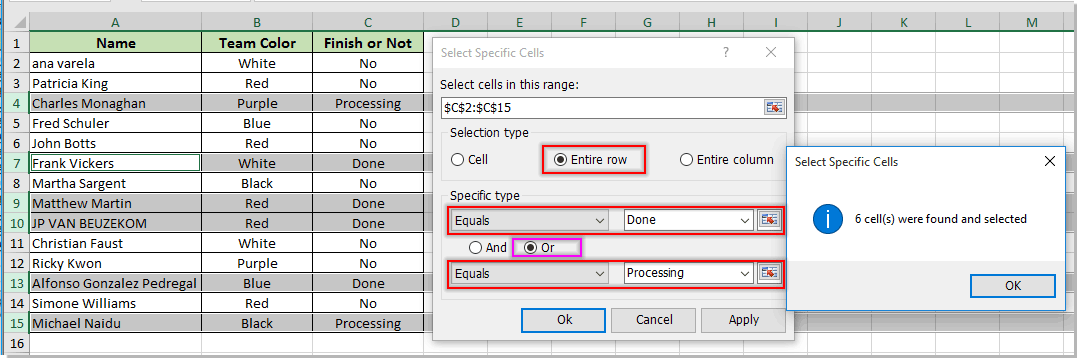If you want to have a free trial ( 30-day) of this utility, please click to download it, and then go to apply the operation according above steps.

### The Best Office Productivity Tools

#### Kutools for Excel Solves Most of Your Problems, and Increases Your Productivity by 80%

• Reuse: Quickly insert complex formulas, charts and anything that you have used before; Encrypt Cells with password; Create Mailing List and send emails...
• Super Formula Bar (easily edit multiple lines of text and formula); Reading Layout (easily read and edit large numbers of cells); Paste to Filtered Range...
• Merge Cells/Rows/Columns without losing Data; Split Cells Content; Combine Duplicate Rows/Columns... Prevent Duplicate Cells; Compare Ranges...
• Select Duplicate or Unique Rows; Select Blank Rows (all cells are empty); Super Find and Fuzzy Find in Many Workbooks; Random Select...
• Exact Copy Multiple Cells without changing formula reference; Auto Create References to Multiple Sheets; Insert Bullets, Check Boxes and more...
• Extract Text, Add Text, Remove by Position, Remove Space; Create and Print Paging Subtotals; Convert Between Cells Content and Comments...
• Super Filter (save and apply filter schemes to other sheets); Advanced Sort by month/week/day, frequency and more; Special Filter by bold, italic...
• Combine Workbooks and WorkSheets; Merge Tables based on key columns; Split Data into Multiple Sheets; Batch Convert xls, xlsx and PDF...
• More than 300 powerful features. Supports Office/Excel 2007-2019 and 365. Supports all languages. Easy deploying in your enterprise or organization. Full features 30-day free trial. 60-day money back guarantee.#### Office Tab Brings Tabbed interface to Office, and Make Your Work Much Easier

• Enable tabbed editing and reading in Word, Excel, PowerPoint, Publisher, Access, Visio and Project.
• Open and create multiple documents in new tabs of the same window, rather than in new windows.
• Increases your productivity by 50%, and reduces hundreds of mouse clicks for you every day!No ratings yet. Be the first to rate!
This comment was minimized by the moderator on the site
Hello, I found this particular guide really helpful over others I've seen. Thank you! The trouble I am having is that if I change my desired value to 'Closed' I have to run F5 to move the row. I would like it to move automatically. I am new to Excel so your assistance is greatly appreciated. Sub Cheezy() Dim xRg As Range Dim xCell As Range Dim I As Long Dim J As Long I = Worksheets("ECR Incident Tracker").UsedRange.Rows.Count J = Worksheets("Resolved Issues").UsedRange.Rows.Count If J = 1 Then If Application.WorksheetFunction.CountA(Worksheets("Resolved Issues").UsedRange) = 0 Then J = 0 End If Set xRg = Worksheets("ECR Incident Tracker").Range("B1:B" & I) On Error Resume Next Application.ScreenUpdating = False For Each xCell In xRg If CStr(xCell.Value) = "Closed" Then xCell.EntireRow.Copy Destination:=Worksheets("Resolved Issues").Range("A" & J + 1) xCell.EntireRow.Delete J = J + 1 End If Next Application.ScreenUpdating = True End Sub
This comment was minimized by the moderator on the site
Hello, I am trying to automate moving the cells over without having to open the module and press F5 as well. Did you ever resolve this question? Thank you in advance!
This comment was minimized by the moderator on the site
Crystal provided information on how to do that today - have a look at page one of this thread to see her response. It automatically moves the row with today's date in a column (L in my case) to a different worksheet.
This comment was minimized by the moderator on the site
I am running this code and am trying to move a row based on today's date appearing in column I - I have changed Range("B1:B" & I) to read Range(I1:I" & I) . I have changed "Done" in your example to Date. However, when today's date appears anywhere in the row, not just in the I column as required, the row moves to the alternate worksheet. Any idea why this is happening and how I can have the row move only when today's date is in column I, regardless of whether today's date appears in other columns?
This comment was minimized by the moderator on the site
If I wanted to have many values and many sheets to move my row to, I would have to write the whole code again with a different value for that cell? Meaning, if I put NA in one cell it goes to Na sheet, and if I put W# it will go to the wrong number sheet etc.
This comment was minimized by the moderator on the site
hi, this was very helpful. Is there a way to do this without having the row of data moved to the second sheet, but rather having it copied? So the data would remain on both sheets?
This comment was minimized by the moderator on the site
Hi the code was very helpful, but instead of the copying the entire row i require the a certain selection of row to be moved to the next sheet. how can i define a range instead of a entire row Sub Cheezy() Dim xRg As Range Dim xCell As Range Dim I As Long Dim J As Long I = Worksheets("Sheet1").UsedRange.Rows.Count J = Worksheets("Sheet2").UsedRange.Rows.Count If J = 1 Then If Application.WorksheetFunction.CountA(Worksheets("Sheet2").UsedRange) = 0 Then J = 0 End If Set xRg = Worksheets("Sheet1").Range("C1:C" & I) On Error Resume Next Application.ScreenUpdating = False For Each xCell In xRg If CStr(xCell.Value) = "Done" Then xCell.EntireRow.Copy Destination:=Worksheets("Sheet2").Range("A" & J + 1) J = J + 1 End If Next Application.ScreenUpdating = True End Sub
This comment was minimized by the moderator on the site
what would be the code if I want to copy rows (specific cells) into another sheet to specific cells? BUT also based upon a value Example: color product images string white blender 2 whiteblender2 black juicer 3 blackjuicer3 red tv 1 redtv1 green iron 4 greeniron4 I would like the string copied to another sheet but the number in the images column tells how many times it should be copied (so, in this case, the blender string should be copied in 2 rows
This comment was minimized by the moderator on the site
Hi, Very nice piece of code, working very well. How to change this code to move rows from one table to another table, instead of one sheet to another sheet? Many thanks !
This comment was minimized by the moderator on the site
Hi I am trying to use the code but I receive a syntax error on  Dim xCell As Range. Can you help please ?
This comment was minimized by the moderator on the site
Sub Cheezy() Dim xRg As Range Dim xCell As Range Dim I As Long Dim J As Long I = Worksheets("Sheet1").UsedRange.Rows.Count J = Worksheets("Sheet2").UsedRange.Rows.Count If J = 1 Then If Application.WorksheetFunction.CountA(Worksheets("Sheet2").UsedRange) = 0 Then J = 0 End If Set xRg = Worksheets("Sheet1").Range("C1:C" & I) On Error Resume Next Application.ScreenUpdating = False For Each xCell In xRg If CStr(xCell.Value) = "Done" Then xCell.EntireRow.Copy Destination:=Worksheets("Sheet2").Range("A" & J + 1) xCell.EntireRow.Delete J = J + 1 End If Next Application.ScreenUpdating = True End Sub how can add a second worksheet to have rows moved to sheet2?
This comment was minimized by the moderator on the site
What should I input if I want to include any date as my value? So the row stays on sheet 1 if it has no date, and moves to sheet 2 if it does?
This comment was minimized by the moderator on the site
[quote]hi, this was very helpful. Is there a way to do this without having the row of data moved to the second sheet, but rather having it copied? So the data would remain on both sheets?By Maddie[/quote] did anybody resolve this
This comment was minimized by the moderator on the site
Remove this "xCell.EntireRow.Delete" from the code
This comment was minimized by the moderator on the site
When I delete that line of code and run the macro again, Excel freezes. Why and how do I fix it?? I want the data to be on both worksheets and not to be deleted from the original. TIA
This comment was minimized by the moderator on the site
is there an answer for this? Mine freezes as well I would like to copy but not delete the row
This comment was minimized by the moderator on the site
Good Day,
The below VBA code can help you to only copy the rows instead of deleting them.

Sub Cheezy()
Dim xRg As Range
Dim xCell As Range
Dim I As Long
Dim J As Long
Dim K As Long
I = Worksheets("Sheet1").UsedRange.Rows.Count
J = Worksheets("Sheet2").UsedRange.Rows.Count
If J = 1 Then
If Application.WorksheetFunction.CountA(Worksheets("Sheet2").UsedRange) = 0 Then J = 0
End If
Set xRg = Worksheets("Sheet1").Range("C1:C" & I)
On Error Resume Next
Application.ScreenUpdating = False
For K = 1 To xRg.Count
If CStr(xRg(K).Value) = "Done" Then
xRg(K).EntireRow.Copy Destination:=Worksheets("Sheet2").Range("A" & J + 1)
J = J + 1
End If
Next
Application.ScreenUpdating = True
End Sub
This comment was minimized by the moderator on the site
Hi, I'm looking for a variation on this. I need the script to run continuously, or failing that whenever the value in that specific field changes. The code itself works but needs to be run independently. I'd like it to be automated. Can anyone assist?

As an aside, if I only want it to copy over specific cells in the range, how is that accomplished?
This comment was minimized by the moderator on the site
Dear Rob,

If you need the script to run automatically when cells in that field changed, the below VBA code can help you. Please right click current sheet (the sheet with rows you will move automatically) tab, then select View Code from the context menu. Then copy and paste the below VBA script into the Code window.

Private Sub Worksheet_Change(ByVal Target As Range)

Dim xCell As Range

Dim I As Long
On Error Resume Next

Application.ScreenUpdating = False

Set xCell = Target(1)
If xCell.Value = "Done" Then
I = Worksheets("Sheet2").UsedRange.Rows.Count
If I = 1 Then

If Application.WorksheetFunction.CountA(Worksheets("Sheet2").UsedRange) = 0 Then I = 0

End If

xCell.EntireRow.Copy Worksheets("Sheet2").Range("A" & I + 1)

xCell.EntireRow.Delete
End If

Application.ScreenUpdating = True

End Sub

For your second question, do you mean just copy several cells instead of the whole row? Or would you please provide a screenshot of your question? Thank you!

Best Regards, Crystal
This comment was minimized by the moderator on the site
Crystal,

Your help is more then needed :)

How we can add another crtieria here, for example i would like to transfer Completed beside Done:

Private Sub Worksheet_Change(ByVal Target As Range)

Dim xCell As Range

Dim I As Long
On Error Resume Next

Application.ScreenUpdating = False

Set xCell = Target(1)
If xCell.Value = "Done" Then
I = Worksheets("Sheet2").UsedRange.Rows.Count
If I = 1 Then

If Application.WorksheetFunction.CountA(Worksheets("Sheet2").UsedRange) = 0 Then I = 0

End If

xCell.EntireRow.Copy Worksheets("Sheet2").Range("A" & I + 1)

xCell.EntireRow.Delete
End If

Application.ScreenUpdating = True

End Sub
This comment was minimized by the moderator on the site
Hi Crystal
This is the most useful info I have found on the web and this macro does what I want. But I am moving the rows from one table to another table - and with this macro the information moves ot the first free line outside of the table, not the next free line in the table? Can you help?
This comment was minimized by the moderator on the site
I am running this code and am trying to move a row based on today's date appearing in column I - I have changed Range("B1:B" & I) to read Range(I1:I" & I) . I have changed "Done" in your example to Date. However, when today's date appears anywhere in the row, not just in the I column as required, the row moves to the alternate worksheet. Any idea why this is happening and how I can have the row move only when today's date is in column I, regardless of whether today's date appears in other columns?
This comment was minimized by the moderator on the site
Dear David,

The code works well for me after changing the range and the variate value to date. The date format in your code must match the date format you used in worksheet. Or is it convenient for you to attach your worksheet?
This comment was minimized by the moderator on the site
Hi Crystal,

I am not clear what you mean when you say that the code and spreadsheet date formats must match - I am not a VB expert, more a novice level. In my spreadsheet I enter today's date in column F as the entry date of the row, in the format ctrl + :. I enter the expiration date in column "I" in the mm/dd/yyyy format. However, this causes problems when making a new row entry and entering today's date in column F because, as soon as it is entered, the row is moved to the new worksheet.Additionally the additional code to run whenever the workbook is opened does not appear to run without me forcing it to do so. Sorry for what might be to you very trivial issues but I just can't get my hear around these issues. Any help would be appreciated.
This comment was minimized by the moderator on the site
Dear David,

I have tried as exactly what you mentioned above, but the issue dose not appear in my case. Can you provide your Excel version? I need more information to help solving this problem. Sorry to trouble you again.

Best Regards, Crystal
This comment was minimized by the moderator on the site
Crystal, these are the worksheets concerned. You will see in the copied code that I am searching for "up to " today's date in column L and if "up to" and including today's date is in that column then I want to move the row containing that date to a new worksheet. Currently, when I enter today's date anywhere in the row(for example column F if a solicitation is issued today) it automatically moves the entire row to the archived spreadsheet. I usually enter today's date by using the ctrl + : combination, usually in column F.
Additionally I would like this move to happen when I open the workbook. Currently I need to go to show code then press F5. Any advice on how to do that would be welcomed.
This comment was minimized by the moderator on the site
Unfortunately my macro enabled workbook will not upload as it says format not supported. These are in Excel 2016
This comment was minimized by the moderator on the site
Dear David,

The following VBA code can help you to achieve it.

Private Sub Workbook_Open()
Dim xRg As Range
Dim xCell As Range
Dim I As Long
Dim J As Long
I = Worksheets("CURRENT OASIS OPPORTUNITIES").UsedRange.Rows.Count
J = Worksheets("ARCHIVED OASIS OPPORTUNITIES").UsedRange.Rows.Count
If J = 1 Then
If Application.WorksheetFunction.CountA(Worksheets("ARCHIVED OASIS OPPORTUNITIES").UsedRange) = 0 Then J = 0
End If
Set xRg = Worksheets("CURRENT OASIS OPPORTUNITIES").Range("L1:L" & I)
On Error Resume Next
Application.ScreenUpdating = False
For Each xCell In xRg
If CStr(xCell.Value) = Date Then
xCell.EntireRow.Copy Destination:=Worksheets("ARCHIVED OASIS OPPORTUNITIES").Range("A" & J + 1)
xCell.EntireRow.Delete
J = J + 1
End If
Next
End Sub

Notes:
1. You need to put the VBA script into the ThisWorkbook code window;
2. Your workbook need to be saved as Excel Macro-Enabled Workbook.

After the above operartion, every time when you open the workbook, an entire row will be moved to ARCHIVED worksheet if cell in column L reaches today's date.

Beast Regards, Crystal
This comment was minimized by the moderator on the site
Thanks Crystal,
This works great if today's date is achieved in the column L. Is there any way to include up to today's date in column L also, so that if I do not check the workbook for a number of days it will automatically include earlier dates prior to today's? Thank you very much for your help.
This comment was minimized by the moderator on the site
Dear David,

Sorry I am not sure I got your question. If so, all rows will be moved as long as earlier dates appear in column L?
This comment was minimized by the moderator on the site
Hi Crystal,

If I don't open my worksheet for a few days and the date entered in column L has now passed, i.e. the date in a cell in column L is September 11, 2017 but don't open my worksheet until September 13, I would like all the entries in column L to be checked for every date up to today's date then move the corresponding rows to the new sheet. Currently with the code you graciously provided, only rows with the current date in column L are moved to the new sheet leaving behind those with an earlier date in column L, which I currently move manually to the new sheet. Thanks for your help.
This comment was minimized by the moderator on the site
Dear David,

I get your point. Please try the below VBA script. When open the workbook, all rows with dates up to today's date in column L will be moved to new specified sheet.

Private Sub Workbook_Open()
Dim xRg As Range
Dim xRgRtn As Range
Dim xCell As Range
Dim xLastRow As Long
Dim I As Long
Dim J As Long
On Error Resume Next
xLastRow = Worksheets("CURRENT OASIS OPPORTUNITIES").UsedRange.Rows.Count
If xLastRow < 1 Then Exit Sub
J = Worksheets("ARCHIVED OASIS OPPORTUNITIES").UsedRange.Rows.Count
If J = 1 Then
If Application.WorksheetFunction.CountA(Worksheets("ARCHIVED OASIS OPPORTUNITIES").UsedRange) = 0 Then J = 0
End If
Set xRg = Worksheets("CURRENT OASIS OPPORTUNITIES").Range("L1:L" & xLastRow)
For I = 2 To xLastRow
If xRg(I).Value > Date Then Exit Sub
If xRg(I).Value <= Date Then
xRg(I).EntireRow.Copy Destination:=Worksheets("ARCHIVED OASIS OPPORTUNITIES").Range("A" & J + 1)
xRg(I).EntireRow.Delete
J = J + 1
I = I - 1
End If
Next
End Sub

You need to put the VBA script into the ThisWorkbook code window and save the workbook as an Excel Macro-Enabled Workbook.
This comment was minimized by the moderator on the site
Thank you Crystal, That works just fine.
This comment was minimized by the moderator on the site
Crystal, I was a bit hasty in responding that the code worked. I opened my workbook today and rows containing prior date entries in column L cell are still in the "current oasis opportunities worksheet" and have not moved to the "archived oasis worksheet" as expected. Any ideas why this would be the case?
This comment was minimized by the moderator on the site
The highlighted cells are in column L in respect of the question above and are the criteria (up to today's date) for moving the row to the new worksheet. Hope this image helps.
This comment was minimized by the moderator on the site
This is also a copy of the VBA window related to the above.
This comment was minimized by the moderator on the site
Crystal, I was a bit hasty in responding that the code worked. I opened my workbook today and rows containing prior date entries in column L cell are still in the "current oasis opportunities worksheet" and have not moved to the "archived oasis worksheet" as expected. Any ideas why this would be the case?
This comment was minimized by the moderator on the site
Crystal,

As I can't upload my workbook I will reproduce the rows & columns here

A B C D E F G H I J K L
# Type Set-Aside Solicitation Amend # Issue Date Questions Customer Delivery Location Project Proposal Due

1 SS SB 1234567 1 09/6/17 No Army Name Place Drive Tank 09/10/17

Using the code below, I want it to move an entire row to a new worksheet when column L reaches today's date. Also if I have not completed the worksheet for a number of days I would like it to use "up to today's date" search in column L to do the same. I would also like it to do this automatically when I open the workbook if possible. Currently if I enter today's date in any cell in the row, for example column F when entering data, the entire row moves to the archive worksheet. (Using Excel 2016)

[Module 1 Code]

Sub DaveV()

Dim xRg As Range

Dim xCell As Range

Dim I As Long

Dim J As Long

I = Worksheets("CURRENT OASIS OPPORTUNITIES").UsedRange.Rows.Count

J = Worksheets("ARCHIVED OASIS OPPORTUNITIES").UsedRange.Rows.Count

If J = 1 Then
If Application.WorksheetFunction.CountA(Worksheets("ARCHIVED OASIS OPPORTUNITIES").UsedRange) = 0 Then J = 0

End If

Set xRg = Worksheets("CURRENT OASIS OPPORTUNITIES").Range("L1:L" & I)

On Error Resume Next

Application.ScreenUpdating = False

For Each xCell In xRg

If CStr(xCell.Value) = Date Then

xCell.EntireRow.Copy Destination:=Worksheets("ARCHIVED OASIS OPPORTUNITIES").Range("A" & J + 1)
xCell.EntireRow.Delete

J = J + 1
End If

Next
Application.ScreenUpdating = True

End Sub
This comment was minimized by the moderator on the site
[Sheet 1 Code]

Private Sub Worksheet_Change(ByVal Target As Range)
Dim xCell As Range
Dim I As Long
On Error Resume Next
Application.ScreenUpdating = False
Set xCell = Target(1)
If xCell.Value = Date Then
I = Worksheets("ARCHIVED OASIS OPPORTUNITIES").UsedRange.Rows.Count
If I = 1 Then
If Application.WorksheetFunction.CountA(Worksheets("ARCHIVED OASIS OPPORTUNITIES").UsedRange) = 0 Then I = 0 End If
xCell.EntireRow.Copy Worksheets("ARCHIVED OASIS OPPORTUNITIES").Range("A" & I + 1)
xCell.EntireRow.Delete
End If
Application.ScreenUpdating = True
End Sub

Hope the above helps but I am not a VBA person therefore do not understand how to make the code do what I need. Your help would be appreciated.
This comment was minimized by the moderator on the site
There is a big error in your script!

Say that you detected that row 7 has the word "Done" in column C, so you copy it and delete the row.
Once you deleted the row, the next row in the list will be row 9 and not 8, because once you removed the 7th line, now the 8th line content is in line 7, and all the lines went up 1 row. So the next row to check was supposed to be row #8, but now it contains the data that previously was on row #9, so every time you are deleting a row, you are actually skipping a row to check!!!
This comment was minimized by the moderator on the site
Dear Shau Alon,

Thank you for your comment. The code has been updated with the error fixed. Thank you so much for your assistant.

Best Regards, Crystal
This comment was minimized by the moderator on the site
I think this is happening to me, it keeps copying the same row over and over even though it says the code was updated. This is what I have:

Sub Cheezy()
'Updated by Kutools for Excel 2017/8/28
Dim xRg As Range
Dim xCell As Range
Dim I As Long
Dim J As Long
Dim K As Long
I = Worksheets("PURCHASE FORCAST").UsedRange.Rows.Count
J = Worksheets("Purchase Archive").UsedRange.Rows.Count
If J = 1 Then
If Application.WorksheetFunction.CountA(Worksheets("Purchase Archive").UsedRange) = 0 Then J = 0
End If
Set xRg = Worksheets("PURCHASE FORCAST").Range("H3:H" & I)
On Error Resume Next
Application.ScreenUpdating = False
For K = 1 To xRg.Count
If CStr(xRg(K).Value) = "Yes" Then
xRg(K).EntireRow.Copy Destination:=Worksheets("Purchase Archive").Range("A" & J + 1)
xRg(K).EntireRow.Delete
If CStr(xRg(K).Value) = "Yes" Then
K = K - 1
End If
J = J + 1
End If
Next
Application.ScreenUpdating = True
End Sub
This comment was minimized by the moderator on the site
Hi Fred,
Each time you run the code, the code searches for the specified range, so it copies the same row over and over again because it can't tell which row has already been copied. To avoid copying the same row repeatedly, you can have the code run automatically when a matching value is entered in the specified cell.
In the worksheet named "PURCHASE FORCAST", right click the sheet tab and click View Code from the context menu. Then copy the following VBA code in the Sheet (Code) window.

``````Private Sub Worksheet_SelectionChange(ByVal Target As Range)
'Updated by Kutools for Excel 20220830
Dim xRg As Range
Dim xCell As Range
Dim I As Long
Dim J As Long
Dim K As Long
I = Worksheets("PURCHASE FORCAST").UsedRange.Rows.Count
J = Worksheets("Purchase Archive").UsedRange.Rows.Count
If J = 1 Then
If Application.WorksheetFunction.CountA(Worksheets("Purchase Archive").UsedRange) = 0 Then J = 0
End If
Set xRg = Worksheets("PURCHASE FORCAST").Range("H3:H" & I)
On Error Resume Next
Application.ScreenUpdating = False
For K = 1 To xRg.Count
If CStr(xRg(K).Value) = "Yes" Then
xRg(K).EntireRow.Copy Destination:=Worksheets("Purchase Archive").Range("A" & J + 1)
xRg(K).EntireRow.Delete
If CStr(xRg(K).Value) = "Yes" Then
K = K - 1
End If
J = J + 1
End If
Next
Application.ScreenUpdating = True
End Sub``````
This comment was minimized by the moderator on the site
Could someone help me make this work? I've tried to change the part that need to match up with my file, but this comes up and I'm not sure what to do.
This comment was minimized by the moderator on the site
it says file not supported when I try to upload the excel file. Sorry...struggling with this today.
This comment was minimized by the moderator on the site
I would like help for a similar task, but slightly different. I have 5 columns of numbers, around 25000 per column, each column with a heading 1-5.I would like to copy the entire row to another sheet if the value of column 1 is great than zero, OR column 2 is greater than zero, OR column 3 is less than zero, OR column 4 is great than five OR column 5 is greater than two etc. is this possible ?
This comment was minimized by the moderator on the site
image upload not working... sorry.
This comment was minimized by the moderator on the site
Hello,
Please use the upload button of this one.
This comment was minimized by the moderator on the site
So the aim is to see if any of the gasses are over a limit which i will set in the formula, the entire roe is COPIED onto a new sheet.

Thank you so much for any help.
This comment was minimized by the moderator on the site
Image attached
This comment was minimized by the moderator on the site
Dear Michael,
Maybe you can solve this probem by using an Excel add-in. Here i recommend you the Select Specific Cells utility of Kutools for Excel. With this utility, you can easily select all rows in a certian range if the value of a specified column is greater than or less than a number. After selecting all needed rows, you can manually copy and paste them to a new worksheet. See below attached image.

https://www.extendoffice.com/product/kutools-for-excel/excel-select-specific-cells-rows.html
This comment was minimized by the moderator on the site
thanks for this formula, but I had a problem which is when I want to move the row to another sheet, it doesn't happen automatically. can you give me another formula ? so whenever I change the value of the cell's, it moved automatic.

thanks
This comment was minimized by the moderator on the site
Dear janang,
The code dose not happen automatically until you trigger the run button manually.
This comment was minimized by the moderator on the site
Hi,

I would like to have this macro set up but with 2 arguments. I managed to get the macro working in my file based on the value of the cells in column O. However I would like for the Macro to check if Column S is filled out (or <> "") as well, before moving the row. Lastly, I would also like to have the copied rows be the same formatting as the rows in the second sheet. Does that change completely the macro?
This comment was minimized by the moderator on the site
Dear Hugues,
I do not know if I understand you the right way. You mean that if cell in column S is filled out and cell in Column O contains the certain value at the same time, then move the row with formatting? Otherwise, don't move?
This comment was minimized by the moderator on the site
Hello Crystal,

Yes that is exactly what I mean. In fact, my data is about projects. My column O is the status of my project, and S the end date of my project.
I want my users, the people that have the information and will need to insert it, to be able to "Archive" a project ONLY if they have their status as "Closed" and they have inserted an "End date".

I hope this helps clarify things
This comment was minimized by the moderator on the site
Dear Hugues,
Sorry for replying so late. The following VBA code can help you solve the problem. Please follow the steps in this article to apply the VBA script.

Sub MoveRowBasedOnCellValue()
Dim xRgStatus As Range
Dim xRgDate As Range
Dim I As Long
Dim J As Long
Dim K As Long
I = Worksheets("Sheet1").UsedRange.Rows.Count
J = Worksheets("Sheet2").UsedRange.Rows.Count
If J = 1 Then
If Application.WorksheetFunction.CountA(Worksheets("Sheet2").UsedRange) = 0 Then J = 0
End If
Set xRgStatus = Worksheets("Sheet1").Range("O1:O" & I)
Set xRgDate = Worksheets("Sheet1").Range("S1:S" & I)
On Error Resume Next
Application.ScreenUpdating = False
Application.CutCopyMode = False
xRgStatus(1).EntireRow.Copy
Worksheets("Sheet2").Range("A" & J + 1).PasteSpecial xlPasteAllUsingSourceTheme
J = J + 1
For K = 2 To xRgStatus.Count
If CStr(xRgStatus(K).Value) = "Closed" Then
If (xRgDate(K).Value <> "") And (TypeName(xRgDate(K).Value) = "Date") Then
xRgStatus(K).EntireRow.Copy
Worksheets("Sheet2").Range("A" & J + 1).PasteSpecial xlPasteAllUsingSourceTheme
J = J + 1
End If
End If
Next
Application.CutCopyMode = True
Application.ScreenUpdating = True
End Sub
This comment was minimized by the moderator on the site
Dear Crystal,

Thank you so much for your help!

Regards,

Hugues
This comment was minimized by the moderator on the site
Hello,

How do I copy the rows instead of moving them?
This comment was minimized by the moderator on the site
Hello,

I know this has been posted a few times but I can't find the answer. How can I copy the material to the new sheet and NOT delete it from the original sheet?
This comment was minimized by the moderator on the site
Dear Mike,
If you want to copy the rows instead of deleting them, the below VBA code can help you. Thank you for your comment!

Sub Cheezy()
Dim xRg As Range
Dim xCell As Range
Dim I As Long
Dim J As Long
Dim K As Long
I = Worksheets("Sheet1").UsedRange.Rows.Count
J = Worksheets("Sheet2").UsedRange.Rows.Count
If J = 1 Then
If Application.WorksheetFunction.CountA(Worksheets("Sheet2").UsedRange) = 0 Then J = 0
End If
Set xRg = Worksheets("Sheet1").Range("C1:C" & I)
On Error Resume Next
Application.ScreenUpdating = False
For K = 1 To xRg.Count
If CStr(xRg(K).Value) = "Done" Then
xRg(K).EntireRow.Copy Destination:=Worksheets("Sheet2").Range("A" & J + 1)
J = J + 1
End If
Next
Application.ScreenUpdating = True
End Sub
This comment was minimized by the moderator on the site
Hi,

I'm new to using macros, is it possible to paste the data below after a certain value and will be repeated until end of column?
Like this:

Transfer "Blue" after "Color"

A1 = Blue
A5= Color
A6= (transfer "Blue" here)
and so on...
This comment was minimized by the moderator on the site
Dear John,
Do you mean if a cell contains "Color" in a column, then copy text of the first cell to the cell below the "Color" one and repeat copy this text until end of the column?
There are no comments posted here yet